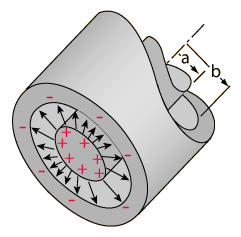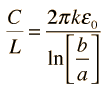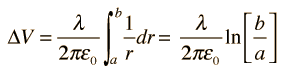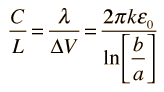# Cylindrical Capacitor

For a cylindrical geometry like a coaxial cable, the capacitance is usually stated as a capacitance per unit length. The charge resides on the outer surface of the inner conductor and the inner wall of the outer conductor. The capacitance expression isShow

For inside radius a = m,
outside radius b = m ,
and dielectric constant k = ,
the capacitance per unit length is
C/L = F/m = x10^ F/m = pF/m
For a length of L = m the capacitance is
C = F = x10^ F = pF

Index

Capacitor Concepts

 HyperPhysics***** Electricity and Magnetism R Nave
Go Back# Cylindrical Capacitor

The capacitance for cylindrical or spherical conductors can be obtained by evaluating the voltage difference between the conductors for a given charge on each. By applying Gauss' law to an infinite cylinder in a vacuum, the electric field outside a charged cylinder is found to beThe voltage between the cylinders can be found by integrating the electric field along a radial line:From the definition of capacitance and including the case where the volume is filled by a dielectric of dielectric constant k, the capacitance per unit length is defined asCalculation
Index

Capacitor Concepts

 HyperPhysics***** Electricity and Magnetism R Nave
Go Back